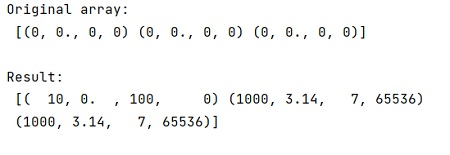# How to return a view of several columns in NumPy structured array in Python?

In this tutorial, we will learn how to return a view of several columns in NumPy structured array in Python? By Pranit Sharma Last updated : April 08, 2023

We know that we can see several columns at once in a numpy structured array by indexing with a list of the field names. But, all them seem to be a copy and not a view.

However, if we only select one column, it is a view. We need to find a way so we can get the view behavior for more than one column at once.

## NumPy structured array: Return a view of several columns

To return a view of several columns in NumPy structured array, we can just create a dtype object containing only the fields that we want, and use numpy.ndarray() to create a view of the original array.

Let us understand with the help of an example,

## Python code to return a view of several columns in NumPy structured array

```# Import numpy
import numpy as np

# Creating an array
arr = np.zeros(3, dtype=[('x', int), ('y', float), ('z', int), ('t', "i8")])

# Display array
print("Original array:\n",arr,"\n")

# defining a function
def fun(arr, fields):
dtype2 = np.dtype({name:arr.dtype.fields[name] for name in fields})
return np.ndarray(arr.shape, dtype2, arr, 0, arr.strides)

# Setting column so that a view is created in arr
v1 = fun(arr, ["x", "z"])
v1 = 10, 100

v2 = fun(arr, ["y", "z"])
v2[1:] = [(3.14, 7)]

v3 = fun(arr, ["x", "t"])
v3[1:] = [(1000, 2**16)]

# Display result
print("Result:\n",arr,"\n")
```

### Output